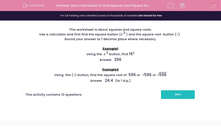# Use a Calculator to Find Squares and Square Roots

In this worksheet, students use a calculator to find the squares and square roots of the given numbers.Key stage:  KS 2

Curriculum topic:   Maths and Numerical Reasoning

Curriculum subtopic:   Square Numbers

Difficulty level:#### Worksheet Overview

This worksheet is about squares and square roots.

Use a calculator and first find the square button (x 2 ) and the square root  button (√)

Example1

Using the  x 2 button, find 162

Example2

Using  the (√) button, find the square root of  596 or  596 or 596